Published: 31 March 2013

# Design and analysis of a novel eddy current damper based on three-dimensional transient analysis

Tian He1
Denghong Xiao2
Xiandong Liu3
Yingchun Shan4
1, 2, 3, 4School of Transportation Science & Engineering, Beihang University, Beijing, 100191, China
Views 1096

#### Abstract

With advantages of no mechanical contact, vacuum compatibility, oil-free, and high reliability, an eddy current damper has a great potential use in space. In this paper, a passive magnetic damper system is developed by using the eddy current damping effect. The proposed eddy current damper utilizes a stationary permanent magnet and two conductive plates, and has significant performance than the model with only one plate. An accurate analytical model based on the electromagnetic theory for this novel eddy current damper is proposed, and the three-dimensional transient analysis based on finite element method is carried out to predict the magnetic field and current density. To optimize the design, simulations are conducted and the design parameters are evaluated from the thickness of magnet and two plates. Simulations for the optimized eddy current damper finite element model are conducted and the damping coefficient as high as 124.079 Ns/m is achieved. The results demonstrate that this novel eddy current damper has high damping performance but simple structure, which is applicable in some vibration isolation systems of spacecraft.

## 1. Introduction

Compared with other types of dampers, such as viscous, viscoelastic, or piezoelectric dampers, the eddy current damper (ECD) has advantages of no mechanical contact, high reliability, high thermal stability, and vacuum compatibility. ECD is the process of subjecting a nonmagnetic conductive material to a time changing magnetic field such that an electromotive force (EMF) is formed. This EMF causes eddy currents to circulate inside the conductor resulting in a magnetic field. The magnetic field formed by these currents then interacts with the applied field to form a force. The density of the currents and the resulting force is dependent on the rate of change of the applied field. In a superconductive material, this force will be permanent; however, due to the internal resistance present in a typical conductor, the current will dissipate into heat and the force will vanish.

For more than two decades, the application of eddy currents for damping purposes has been investigated, including magnetic braking systems, vibration control of rotary machinery, and structural vibration suppression. Wiederick et al.  proposed a simple experiment describing the magnetic braking effect in a thin metal strip and the retarding action on a spinning aluminum disc. MacLatchy et al.  analyzed the falling magnet in a vertical copper pipe, integrating the eddy current over the pipe’s length to find the retarding force on the magnet. Cadwell  investigated the braking force exerted on an aluminum plate as it passed between the poles of a horseshoe electromagnet. Lee and Park  had developed a model for an eddy current braking system that allows for an analytical solution to the problem. Anwar and Stevenson  introduced an enhanced parametric model for a copper-layered eddy current electric machine based on the results from a detailed electromagnetic finite element analysis. Choi  reported on analytical magnetic torque calculations and experimental tests of a radial flux permanent magnet type eddy current brake. Lin et al.  introduced an ECD to suppress the flexural suspension mechanism in a precision positioning stage. Zuo et al.  presented the design and analysis of an ECD. The magnetic field was split into multiple ones with alternating directions so as to reduce the electrical resistance of the eddy current loops and increase the damping force and damping coefficient. Sodano and Bae  reviewed the research into various types of eddy current damping mechanisms and discussed the future of eddy currents with some potential uses. More applications and developments can be seen in the paper.

Although there are those locations that are particularly well suited for ECDs, but perhaps the most promising is in space. Because it does not require any liquid that could leak during operation, had low friction (because of its non-contact nature, no friction is present from the damper), and provided small variation in damping over a fairly wide range of temperatures. When a device is placed into orbit, the system must function for its entire lifespan without requiring any type of maintenance. This can place limitations on the type of damper used, leaving few systems left. The extremely cold temperatures that are present in space actually improve the damping performance of the ECD, due to the decrease in resistivity of the conductor. The advantages listed above provide a combination of attributes that are not available in other damping mechanisms. When a device is placed into orbit, the system must function for its entire lifespan without requiring any type of maintenance.

Gunter et al.  investigated the design of an eddy current damping system for the cryogenic pumps used to deliver the liquid fuel to the main engines of the space shuttle. They stated that the damping generated by the system was sufficient to help suppress the rotor vibration. Cunningham  studied the use of ECDs to suppress the lateral vibration experienced by the cryogenic turbo machinery used in space shuttles. Kienholtz et al.  investigated a magnetic damping system for use in space. They focused on the development of a vibration isolation system to protect a large optical instrument intended for the Hubble telescope from the harsh vibrations experienced during shuttle launch that may damage the sensitive equipment. The isolation system used eight telescoping struts consisting of a titanium coil spring and a passive damper. It was expected that through the use of magnetic dampers the isolation system would have a maintenance-free life of 20 years. However, it had disadvantages of large mass and packing size.

Sodano et al.  proposed an ECD suppressing the transverse vibration of a beam structure, consisting of a single permanent magnet located a small distance from a conductor attached to the beam, which was shown in Fig. 1. As the beam vibrated, the conductor moved perpendicularly to the face of the magnet causing eddy currents to be generated by the radial magnetic flux. This interaction of the magnet, conductor, and beam was modeled using electromagnetic field theory and experiments were performed to validate the accuracy of the model. Additionally, these tests demonstrated that the damper could induce damping levels as high as 35 % of critical. Sodano et al.  also investigated this ECD in both ambient and vacuum conditions to identify the accuracy of the model and to show the functionality of the ECD at vacuum pressure. It was found that the ECD could indeed suppress the vibration of the membrane and that with the ECD; the damping ratio of the membrane was increased to 0.3 at ambient pressure and 0.25 at vacuum pressure.

Fig. 1Configurations of Sodano’s ECD model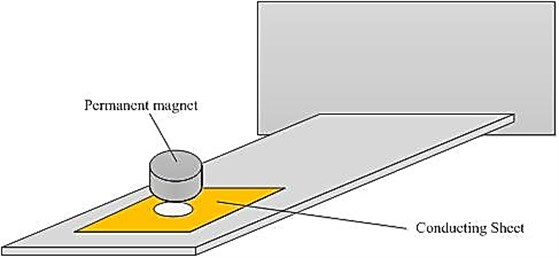By arranging the magnet this way, the radial magnetic flux was used to generate the EMF rather than the axial magnetic flux. Additionally, since the permanent magnet was not attached to the beam, the uncontrolled dynamic characteristics, other than the additional damping to the beam, were unaffected by the damper. The damper was non-contact, and therefore did not cause mass loading or additional stiffness, and avoid local deformation, which was an ideal choice for a damper used with membranes, and it was extensive used in space shuttle.

However, in the previous study only one copper plate was used. In the present study, it is realized that as the magnetic field is distributed symmetrically on both sides of a strip permanent magnet, another copper plate can be placed on the other side of the magnet fixed in the proposed damper proposed by Sodano et al. , as well as to take full advantage of the permanent magnets on both sides of the magnetic field. Building on the this damping system, when two similar copper plates are placed on both sides of a strip permanent magnet, more magnetic flux will be contributed to the eddy current in that two copper plates, causing the damping force in the axial direction to be enhanced as shown in Fig. 2. The increased flux in the radial direction causes the magnetic damper to be far more effective than the use of a single copper plate.

Fig. 2Schematic of the magnetic flux of two copper plates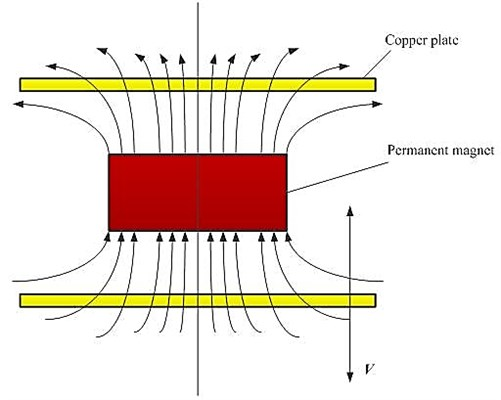This paper employed the eddy current damping effect in the development of a scaled-down damper for axial vibration control applications in space. In addition, a full-size design and evaluation for an ECD applicable to suspension systems in space was introduced. Fig. 3 depicts the configuration of our eddy current damping system, which consisted of a permanent magnet, two copper plates, a mover and an iron shell. The two copper conducting plates connected by a connecting rod with non-magnetic material are located in the magnetic field generated by a single rectangle permanent magnet.

As the ECD has a considerable number of advantages in comparison with other damping devices, a number of applied studies have been conducted on it. For instance, in the previous published works, almost all the fundamental characteristics of the ECD are investigated by analytical approaches. An accurate, analytical model of each system is obtained by applying electromagnetic theory to estimate the electromagnetic forces induced in the system. However, there are some limitations existing in analytical approaches. As in these reports, the attenuation coefficient is not considered to be affected by the eddy current in a conductor in a magnetic field, or by the fringing flux, the shape, or the nonlinear magnetic characteristics of the material. Furthermore, as the damping characteristics are determined by magnetic field distribution of ECD, which is affected by various factors, including magnetic shielding, movement of the metal plate, magnet size, it is difficult to obtain the accurate analytical formulations of ECDs with complex configuration as well as in optimization design. In order to design an ECD as effectively as possible, the above factors should be considered in the numerical analysis, and simulation can be done using finite element analysis. Presently the finite element method (FEM) has been applied in numerical analysis of the magnetic field and the eddy current distributions to investigate ECDs, and several papers have been published on this topic in literature. The results from finite element (FE) analysis and analytical models are verified by experimental studies. Albertz et al.  studied the active eddy current braking system of a high velocity train using the FEM. Kurz et al.  examined the transient behavior of an electromagnetic levitation system consisting of an aluminum plate above two concentric current-carrying coils. Baranski et al.  presented the special software for transient FE analysis of coupled electromagnetic-thermal problems in a squirrel cage induction motor. Laborenz et al.  performed a transient electromagnetic finite element analysis of the eddy current damping device and the resulting damping forces are compared to their analytical solution. Zuo et al.  presented the design and analysis of a new configuration of ECDs, taking into account the influence of the movement of conductors.

Fig. 3Configuration of the proposed ECD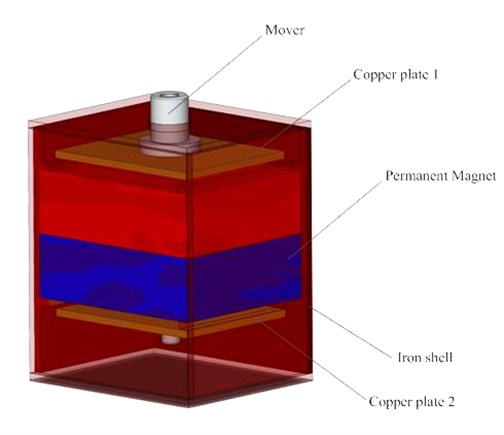Therefore, the main objective of this work was the development to an accurate electro-magnetic formation of a rapid structural design and optimization method for the new ECD based on FEM, which could also be used to simulate and analyze the fundamental characteristics of some novel ECDs with complex structures to verify the validity. In this manuscript a new configuration of ECD was developed based on Sodano model. An analytical model for this ECD was derived based on electromagnetic theory. The magnetic flux generated by a rectangular permanent magnet would be calculated, allowing the eddy current density to be determined. In addition to the analytical modeling, we used FEM to more accurately investigate the damping properties of the ECD by taking account of the edge effects of the finite-size conductors and the non-uniform distribution of magnetic field. Herein, a commercial FE software package, ANSYS, was used to carry out the simulations. The transient electromagnetic finite element analysis of the eddy current damping device was performed, and the resulting damping forces were compared to analytical solution. In the analyses presented in this paper, the electromagnetic field calculations were carried out separately from structural mechanics in a transient analysis in the time domain.

## 2. Description of the prototype and analytical modeling

According to the requirements of layout constraints, the schematic cross-section configuration of the proposed ECD was depicted in Fig. 4, where the two copper plates were the source of the eddy currents due to the movement of the mover which combined them through a rod. Its dimensions were listed in Table 1.

Fig. 4Schematic sketch of damping element consisting of permanent magnet and copper plates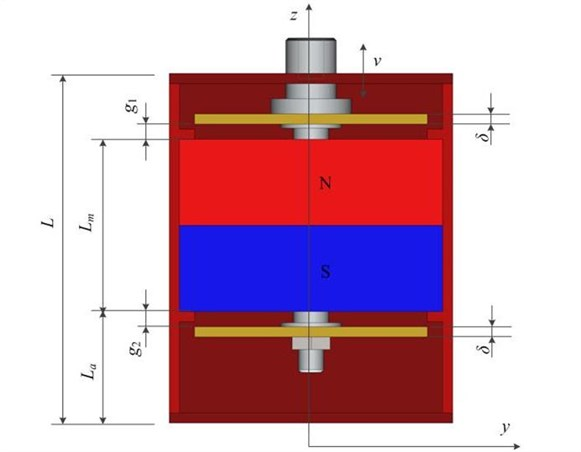Table 1ECD prototype dimensions

 Item/ symbol Value/ unit Magnet material NdFeB alloy ($Br=$ 1.2289 T) Plate and conductor material copper Magnet length ($a$) 52 mm Magnet thickness ($h$) 34 mm Magnet width (${L}_{m}$) 44 mm Iron shell thickness 2 mm Copper plate thickness ($\delta$) 2 mm Copper plate length 46 mm Copper plate width 38 mm Electrical conductivity of copper 5.96×107/$\mathrm{\Omega }$ m Young's modulus of copper 1.08×1011 Pa Air gap (${g}_{1}$) 3 mm Air gap (${g}_{2}$) 3 mm Length of bottom space (${L}_{a}$) 20 mm Length of iron shell ($L$) 69 mm

Fig. 4 showed two conducting sheet of thickness $\delta$ and conductivity moving with velocity $v$ in the air gap ${g}_{1}$ and ${g}_{2}$ of a rectangle magnet. Due to the permanent magnet, a magnetic field was generated in the vertical ($z$) and horizontal ($x$ and $y$) axes. When the copper plate surface was deflected and set in motion in the static magnetic field, an electric field was generated in the conducting sheet. Since the deflection of copper plate was in the vertical direction, the vertical component of the magnetic field does not contribute to the generation of eddy currents. Hence, the electric field on the conductor was dependent on the horizontal component of the magnetic field. As shown in Fig. 4, the eddy currents would circulate on the conducting sheet in the $x-y$ plane, causing a magnetic field to be generated.

The distribution of magnetic flux density of ECD was further studied using the FEM. Both the two-dimensional model (2D) and three-dimensional model (3D) of the damper are simulated, for further analysis of the proposed ECD and to estimate the magnetic flux density. Fig. 5 showed the magnetic flux density analysis results of the proposed mover configuration. Magnetic flux density streamlines and the induced magnetic field were plotted in these two figures.

Fig. 5FE simulation results of the prototype ECD: a) 2D Flux lines plot of the magnetic flux density; b) 3D vector plot of the magnetic flux density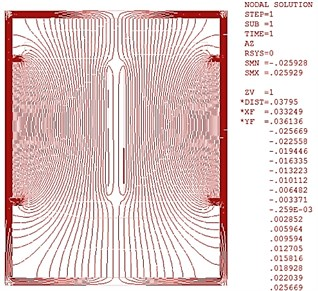a)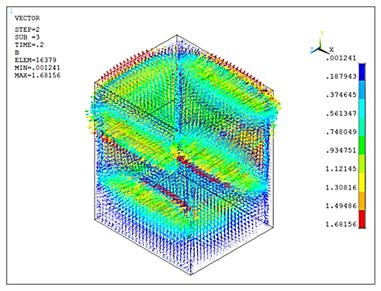b)

Fig. 5(a) demonstrated 2D finite element model of the proposed system. The streamlines represented the magnetic flux density. The 3D vector plot of the magnetic flux density was displayed in Fig. 5(b). It was observed that the rectangle magnetic flux density was concentrated and enhanced at the iron shell, which would affect the current induction. And the magnetic flux density in areas beyond the ECD was exceedingly weak. The influence of electromagnetic leakage could be ignored. To obtain a closed form solution for the damping force, it’s necessary to establish analytical model of the ECD device.

### 2.1.1. Expression of magnetic field distribution of rectangular permanent magnet

To determine the damping force induced on the beam, the magnetic flux $B$ must be calculated at first. As it is difficult to know the exact distribution of permanent magnet, many numerical methods are developed. Gou  derived a set of analytic expressions for magnetic field distributions based on molecular current model.

A rectangular permanent magnet with length $a$, width $b$ and thickness $h$, was magnetized sufficiently in one direction and saturated, which was shown in Fig. 6. The magnetic field at an arbitrary point in the space out of the magnet was produced by all the molecular current of the magnet. As the result of magnetized uniformly, there not only existed the molecular current but the surface current in the magnet. It was reasonable that only the surface loop $ABCD$ current contributes to the magnetic field. It assumed that the current strength in the loop was $I$, so the current density in the plane was parallel to the plane x-y, and $J=I/h$. Denoting a point in the magnet as $\left({x}_{0},{y}_{0},{z}_{0}\right)$ and an arbitrary point in the space out of the magnet as $P\left(x,y,z\right)$, a thin layer between the planes was used, which was shown in Fig. 6. In this thin layer, the current loop was denoted as ${A}^{\text{'}}{B}^{\text{'}}{C}^{\text{'}}D\text{'}$, as $dB$, the magnetic field at point $P\left(x,y,z\right)$, produced by the magnet could be written as follows:

1
$\mathbit{B}={B}_{x}\mathbit{i}+{B}_{y}\mathbit{j}+{B}_{z}\mathbit{k}={\int }_{0}^{h}\left(d{B}_{x}\mathbit{i}+d{B}_{y}\mathbit{j}+d{B}_{z}\mathbit{k}\right),$

where $d{B}_{x}$, $d{B}_{y}$, $d{B}_{z}$were the magnetic induction components in the direction of $x$, $y$, and $z$ at the point $P\left(x,y,z\right)$ produced by current loop ${A}^{\text{'}}{B}^{\text{'}}{C}^{\text{'}}D\text{'}$, which were composed of four sections of $A\text{'}B\text{'}$, $B\text{'}C\text{'}$, ${C}^{\text{'}}{D}^{\text{'}}$ and ${D}^{\text{'}}{A}^{\text{'}}$.

The magnetic flux density due to a circular magnetized strip, shown in Fig. 6, could be written as follows, while the specific calculation process was listed in the Appendix:

2
$\begin{array}{l}{B}_{x}={\int }_{0}^{h}d{B}_{x}=-\frac{K}{2}\left[\Gamma \left(a-x,y,z\right)+\Gamma \left(a-x,b-y,z\right)-\Gamma \left(x,y,z\right)-\Gamma \left(x,b-y,z\right)\right]{|}_{0}^{h},\\ {B}_{y}={\int }_{0}^{h}d{B}_{y}=-\frac{K}{2}\left[\Gamma \left(b-y,x,z\right)+\Gamma \left(b-y,a-x,z\right)-\Gamma \left(x,y,z\right)-\Gamma \left(y,a-x,z\right)\right]{|}_{0}^{h},\\ {B}_{z}={\int }_{0}^{h}d{B}_{z}=-K\left[\begin{array}{c}\varphi \left(y,a-x,z\right)+\varphi \left(b-y,a-x,z\right)+\varphi \left(x,b-y,z\right)+\varphi \left(a-x,b-y,z\right)\\ +\varphi \left(b-y,x,z\right)+\varphi \left(y,x,z\right)+\varphi \left(a-x,y,z\right)+\varphi \left(x,y,z\right)\mathrm{}\end{array}\right]{|}_{0}^{h}\end{array}$

where $\left[\right]{|}_{0}^{h}$ denoted the subtraction between the value of the function $\left[\right]$ at ${z}_{0}=h$ and at ${z}_{0}=0$; $\Gamma$ and $\varphi$ were both a function notation about some independent variables, and the specific expressions were listed as follow:

3
$\Gamma \left({\gamma }_{1},{\gamma }_{2},{\gamma }_{3}\right)=ln\frac{\sqrt{{\gamma }_{1}^{2}+{\gamma }_{2}^{2}+\left({\gamma }_{3}-{z}_{0}{\right)}^{2}}-{\gamma }_{2}}{\sqrt{{\gamma }_{1}^{2}+{\gamma }_{2}^{2}+\left({\gamma }_{3}-{z}_{0}{\right)}^{2}}+{\gamma }_{2}},$
4
$\varphi \left({\phi }_{1},{\phi }_{2},{\phi }_{3}\right)=\left\{\begin{array}{ll}\mathit{arctan}\left(\frac{{\phi }_{1}}{{\phi }_{2}}\frac{{\phi }_{3}-{z}_{0}}{\sqrt{{\phi }_{1}^{2}+{\phi }_{2}^{2}+{\left({\phi }_{3}-{z}_{0}\right)}^{2}}}\right)& \left(y\ne 0\right),\\ 0& \left(y=0\right).\end{array}\right\$

Therefore, it was obvious that we could obtain the magnetic field distribution at an arbitrary point $P\left(x,y,z\right)$ in the space out of the permanent.

Fig. 6Analytical model of the rectangular permanent magnet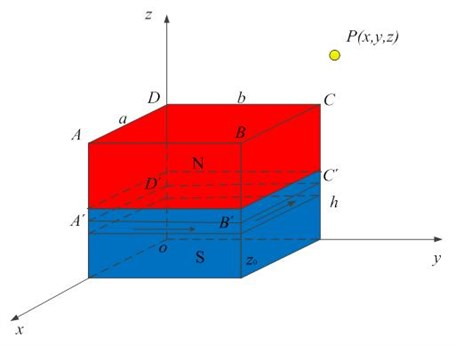### 2.1.2. Eddy current damping force

As shown in Fig. 7, when the two copper plates were moving at a speed of 0.1 m/s, the magnetic field in the axial ($x$), axial ($y$) and axial ($z$) directions around the plates were time-varying due to the relative movement.

Fig. 72D simulation of the ECD, where the air gap g1 is decreased from: a) 3 mm to b) 1 mm; the streamlines represent the magnetic flux density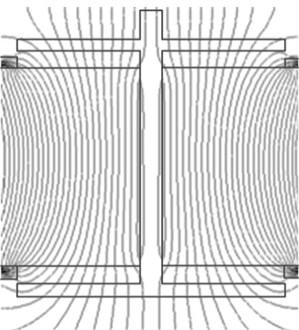a)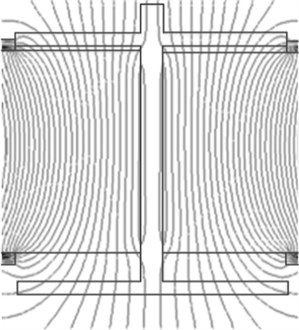b)

The total generated EMF of the copper plates was based on either a time-varying magnetic field, or the relative motion of the conducting plate. The former contribution was associated with the “transformer EMF” and was easily obtained from the third Maxwell equation (Faraday’s law), whereas the latter was associated with the ‘‘motional EMF’’ and was derived from the Lorentz force law by calculating:

5
$V={V}_{trans}+{V}_{motional}=-\underset{s}{\int }\frac{\partial \mathbit{B}}{\partial t}ds+\underset{c}{\int }\left(\mathbit{v}×\mathbit{B}\right)dl.$

And the dampering forces caused by the two copper plates also contained two parts, which were calculated as:

6
$\mathbit{F}={\mathbit{F}}_{trans}+{\mathbit{F}}_{motional}.$

If the surface charges were assumed to be ignored, the current density $J$ induced in the conducting sheet moving in the vertical direction was given by:

7
$\mathbit{J}=\sigma \left(\mathbit{v}×\mathbit{B}\right),$

where the $\mathbit{v}×\mathbit{B}$ term is an electromotive force driving the eddy currents $J$.

Since the velocity of the conducting sheet was in the $z$ direction, the magnetic flux density ${B}_{z}$ did not contribute to the damping force. Using Eqs. (5) and (7), the damping force of a copper plate due to the eddy current was defined by:

8
${\mathbit{F}}_{motional}={\int }_{V}\mathbit{J}×\mathbit{B}dv=-k\sigma hv{\int }_{0}^{a}{\int }_{0}^{b}\sqrt{{x}^{2}+{y}^{2}}\left({B}_{x}^{2}\left(x,y,{l}_{g}\right)+{B}_{y}^{2}\left(x,y,{l}_{g}\right)\right)dxdy,$

where $\delta$ and $v$ were the thickness and the vertical velocity of the conducting sheet, respectively, and ${l}_{g}$ was the distance between the conducting sheet and the bottom of the magnet as shown in Fig. 4, such as ${g}_{1}$ and ${g}_{2}$. Considering the two copper plates in our novel ECD, the damping force due to the eddy current caused by the relative motion of the conducting plate could be calculated as follows:

9
${\mathbit{F}}_{motional}={\mathbit{F}}_{upper-motional}+{\mathbit{F}}_{down-motional}={\int }_{V}\mathbit{J}×\mathbit{B}dv=-k\sigma hv{\int }_{0}^{a}{\int }_{0}^{b}\sqrt{{x}^{2}+{y}^{2}}\left({B}_{x}^{2}\left(x,y,{g}_{1}\right)+{B}_{y}^{2}\left(x,y,{g}_{1}\right)\right)dxdy+\mathrm{}\left(-k\sigma hv{\int }_{0}^{a}{\int }_{0}^{b}\sqrt{{x}^{2}+{y}^{2}}\left({B}_{x}^{2}\left(x,y,{g}_{2}\right)+{B}_{y}^{2}\left(x,y,{g}_{2}\right)\right)dxdy\right).$

Since the magnetic field was not uniform in the vertical direction, the copper plate was prone to a magnetic flux density variation during the reciprocating oscillation. Consequently, the transformer term should be considered in the eddy current calculations. The transformer EMF was calculated by conducting numerical integration over the surface of the conductor plate as follows:

10
${V}_{trans}=-\underset{s}{\int }\frac{\partial \mathbit{B}}{\partial t}ds=-\underset{s}{\int }\left(\frac{\partial \mathbit{B}}{\partial x}\frac{\partial x}{\partial t}+\frac{\partial \mathbit{B}}{\partial y}\frac{\partial y}{\partial t}+\frac{\partial \mathbit{B}}{\partial z}\frac{\partial z}{\partial t}\right)ds,$

where the first and second terms in the integral were zero, by considering the symmetry of the plane flux density about the plate center axis. Therefore, the damping force generated by the transformer eddy currents, was calculated from the respective EMF $\left({V}_{trans}\right)$ as follows:

11
${F}_{trans}=-\frac{k\sigma {v}_{z}}{\sqrt{{x}^{2}+{y}^{2}}}\underset{s}{\int }\frac{\partial {B}_{z}}{\partial t}ds\underset{s}{\int }\left(x{B}_{x}\left(x,y,{l}_{g}\right)+y{B}_{y}\left(x,y,{l}_{g}\right)\right)dV.$

Then like the damping force of a copper plate defined by the motional EMF, the damping force generated by the transformer eddy currents was calculated as:

12
${F}_{trans}={F}_{upper-trans}+{F}_{down-trans}=-\frac{k\sigma {v}_{z}}{\sqrt{{x}^{2}+{y}^{2}}}\underset{s}{\int }\frac{\partial {B}_{z}}{\partial t}ds\underset{s}{\int }\left(x{B}_{x}\left(x,y,{g}_{1}\right)+y{B}_{y}\left(x,y,{g}_{1}\right)\right)dV-\frac{k\sigma {v}_{z}}{\sqrt{{x}^{2}+{y}^{2}}}\underset{s}{\int }\frac{\partial {B}_{z}}{\partial t}ds\underset{s}{\int }\left(x{B}_{x}\left(x,y,{g}_{2}\right)+y{B}_{y}\left(x,y,{g}_{2}\right)\right)dV.$

Taking Eqs. (2), (3) and (4) into Eqs. (9) and (12), the damping force of two copper plates can be calculated. Because it was too difficult to integrate Eq. (9) and (12) analytically, a numerical integration method was used to obtain the damping force. However, Graves et al.  used an equivalent circuit technique to analyze rectangular and circular eddy current damping systems for both types of EMF generation. They found that in almost all realistic situations, the motional EMF devices will have a larger efficiency than the transformer EMF devices. However, for the case of a transformer EMF device with a circular core, it was found that the maximum device efficiency could be made to be approximately one-third greater than that of motional EMF devices. Ebrahimi  had verified that the transformer eddy current contribution was 25 % less than that of the motional eddy currents of a eddy current damping system, which containing an aluminum plate and a permanent magnet in circularity shape. Taking these two examples into consideration, it found that the attribution of transformer EMF in damping force could be ignored to decrease calculation cost and eliminate errors.

## 3. Finite element analysis

### 3.1. Choosing between analysis methods

Applying FEM to the analysis of the eddy current brake involves several choices: choosing between 2D and 3D analysis; choosing between analysis methods. In the past, most FE analyses of eddy current brakes published used 2D analysis. The use of only two dimensions yielded a very limited number of nodes, which made computations easy and fast. However, 2D analysis didn’t account for 3D effects directly and approximations must be made to account for these. The accuracy of the solution suffered greatly. The 2D methods were typically better suited for the analysis of laminated geometries with well-defined current paths, where end effects were either negligible or easily accounted for like electric motors or transformers. Three-dimensional methods, on the other hand, were better suited for geometries where end effects are non-negligible and where current paths were ill-defined such as eddy current brakes in particular and eddy current problems in general. However, the 3D analysis required a powerful processor and a large memory and takes much longer than 2D analysis to reach a solution. This was the result of a much larger number of nodes to be solved.

The 3D FE analysis method was also adopted to predict the eddy current density for conductors moving inside the magnetic field. This is somewhat tricky in ANSYS since moving conductor analysis can be done directly in a 2D static magnetic analysis, but not in 3D. To add conductor velocity, there are two methods suited for the analysis of ECD: 3D harmonic analysis and transient methods.

3D harmonic analysis method is used to simulate a moving conductor under a static magnetic field excitation. As permanent magnet cannot be analyzed for moving conductor during harmonic analysis process, the external field is applied at the exterior plane by applying a nonzero value of the scalar potential based on the equation:

13
${B}_{y}=\mu {H}_{y}=-\mu grad\left(\varphi \right)=-\mu \frac{\Delta \varphi }{\Delta y}.$

With this relationship, the scalar potential value at $\mathrm{\Delta }y$ is:

14
${\varphi }_{y=\mathrm{\Delta }y}=-{B}_{y}\frac{\mathrm{\Delta }y}{{\mu }_{0}}.$

The external magnetic field, whose intensity value is based on 3D static magnetic field analysis, is applied in the area of the conductor plane. A very low frequency less than 0.0001 Hz in the harmonic analysis is employed to closely approximate the static field, and the specific velocity can be assigned to the conductor.

3D transient analysis method starts with a disc at standstill, step up the speed to the desired value, and solve the problem for several time steps until a permanent regime has been reached. As permanent magnet can be considered directly in the 3D transient analysis method, this allows studying the actual transients occurring in a damper and can be applied to geometries varying in the direction of motion.

### 3.2. Verification of the 3D transient analysis method

Zuo et al.  investigated an ECD with 3D harmonic analysis method, and the configuration was shown in Fig. 8. During 3D harmonic analysis the external magnetic field, whose intensity values at each node was based on 3D static magnetic field analysis. And then experiments were conducted to investigate the quasi static and dynamic damping properties of proposed ECD, and the results were compared with the ones from the analytical model. The predictions of FE analysis agreed reasonably well with experimental studies. To the best of the authors’ knowledge, the application of ECD with 3D transient analysis methods has not been addressed in prior publications.

Fig. 8Zuo’s magnetic ECD: a) assemblies of magnetic array and conductor plates and b) top view of magnet array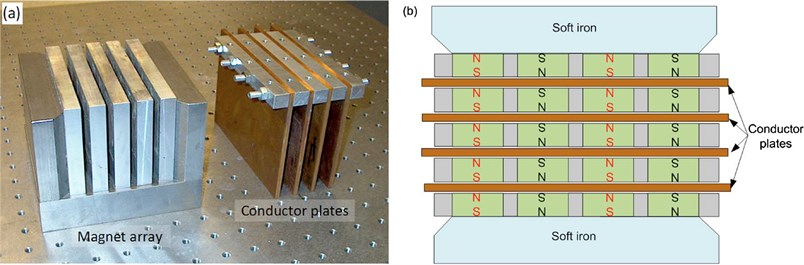In order to verify 3D transient analysis method, Zuo’s ECD model was used. The magnetic and electrical properties of the material can both be defined as linear and isotropic. ANSYS does not allow defining a temperature dependence of the properties for transient method. Consequently, the electrical conductivity of the material is simply defined as isotropic and constant. The time step for the transient analysis was 2 ms, which allowed nodes on either side of the sliding surface to be matched at every time step. Fig. 9 showed the results of the transient analysis of Zuo’s ECD model, when the conductors were moving with a constant velocity.

In Fig. 9(a), the directions of magnetic field in same row were contrary to neighbor with alternative pole directions. The vector display of eddy current in the conductor was shown in Fig. 9(b), and we saw that the eddy current density of the conductor with alternating magnetic poles was significantly higher and there were three loops.

Fig. 9Transient analysis of Zuo’s ECD model: a) magnetic field distribution of the copper plates, b) eddy current distribution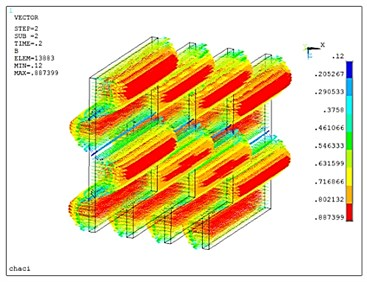a)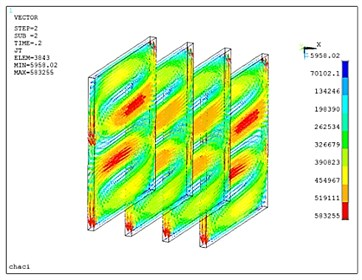b)

The results of analytical model, FEM, and experimental results were compared in Table 2. The 3D harmonic analysis method predicted 2810 Ns/m, which was 7.3 % lower than the experimental result. The 3D transient analysis method predicted 1986.6 Ns/m, which was 10.83 % lower than the experimental result. That’s to say the 3D transient analysis method could also be well used to predict ECD performance. In configuration of our new novel magnetic damper with two copper plates, the horizontal magnetic flux was used to generate the EMF rather than the axial magnetic flux as explained previous. And it was difficult to obtain the value of horizontal magnetic flux density as magnetic flux in axial direction dominates the whole. However, as permanent magnet can be considered directly in the 3D transient analysis method, two copper plates damper model will be solved precisely.

Table 2Results of comparison of the damping coefficients

 Item Damping coefficient (N s/m) Error (%) Loop experiment 2228 Analytical prediction 2810 +26 3D harmonic analysis 2065.4 –7.3 3D transient analysis 1986.6 –10.83

### 3.3. 3D transient analysis of the new novel damper

In order to calculate the damping force more accurately, various significant analysis parameters were discussed in detail. The model was constructed according to the dimensions listed in Table 1.

(1) Mesh quality, density, and time step

Both hexahedral and tetrahedral element types were implemented to mesh the damper model, whose finite elements were shown in Fig. 10 and Fig. 11 respectively. The iteration process of the magnetic field intensity for both hexahedral and tetrahedral element models were plotted when the conductors were moving at a constant velocity. It was clearly seen that several oscillations were occurred in Fig. 11(b), which was mainly due to the poor mesh quality (coarse elements, massive tetrahedral elements, excessive deformation).

Fig. 10Hexahedral element model and its transient analysis: a) hexahedral element model, b) magnetic field intensity of a node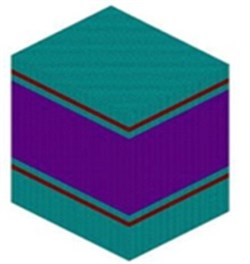a)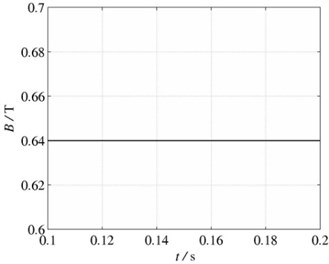b)

Fig. 11Tetrahedral element model and its transient analysis: a) tetrahedral element model, b) magnetic field intensity of a node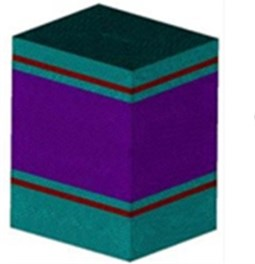a)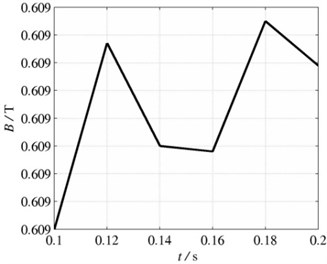b)

The results of analytical models with different element type, element length, and integration step were compared in Table 3. Some significant conclusions could be conducted through the analysis results. When the element length was small enough and integration step was extraordinary short, less difference was between tetrahedral and hexahedral models. It was considered that an adequate mesh density was obtained according to calculation accuracy and cost.

Table 3Effect of mesh quality, density, and time step

 Element length (mm) 2 4 Integration step (s) 0.002 0.005 0.002 0.005 Damping force of hexahedral model (N) 5.35679 5.35444 5.30591 5.3148591 Damping force of tetrahedral model (N) 5.47479 5.47323 5.40975 5.40992

(2) Element density of copper plate

The meshing of the copper plate was of particular importance due to skin effect, which increased the current density in the immediate vicinity of the air-gap surface. If the number of elements in this region was too low, the evolution of the current density profile would be incorrectly computed and incorrect. Table 4 listed the damping force calculation results of three models with different element densities (the number of element layer in the thickness direction of the plate). As seen in Table 4, the number of element layer had little effect on the calculation accuracy, and this parameter could be set as 2.

Table 4Calculation results of different element layer numbers

 Element layer number Damping force (N) 2 5.35679 3 5.35658 4 5.35613

Since the effect of main factors was determined (hexahedral element, 2 mm in element length, 0.002 s in integration step, and 2 layer for element density of copper plate), 3D transient analysis of the new novel ECD was carried out, which was shown in Fig. 12.

We also calculated the damping coefficient using the analytical equation. The damping force calculation result of this new developed prototype based on 3D transient analysis was listed in Table 5. The 3D harmonic analysis method predicted 5.35679 N, which was 5.74 % smaller than the analytical result, since the effect of iron shell was ignored in the analytical prediction.

Table 5Damping force calculation results

 Damping force calculation result (N) Plate 1 Plate 2 Whole damper Error (%) Analytical prediction 2.8415 2.8415 5.683 3D transient analysis 2.09482 3.26197 5.35679 -5.74%

Taking those analysis results into consideration, some significant conclusions can be deduced.

1) Magnetic field distribution of the air was exhibited in Fig. 12(a). It was observed that magnetic field intensity was extremely weak, 0.158E-5T, indicating that the iron shell played an important role in electromagnetic shielding.

2) Fig. 12(c) signified the eddy current distribution of the two copper plates. It was observed that the eddy current in the copper plate 2 was stronger than on the copper plate 1, and more intensive. This was due to the magnetic field in the copper plate 2 was stronger and more uneven then the upper one, leading to a flux change, and thus had a greater eddy current. In addition, according to Lorentz force distribution of the two copper plates as shown in Fig. 12(d), damping force of plate 2 was larger than plate 1, and the specific values were listed in Table 5.

3) The Lorentz forces of the connecting rod between two copper plates were extremely weak, and it had little contribution to the overall damping force, as shown in Fig. 12(e). Also the eddy current was disorganized with tiny value. So in the subsequent analysis of electromagnetic field simulation, the connecting rod can be simplified. This can reduce the complexity of the model, and obtain higher-quality mesh to improve the finite element analysis accuracy.

Fig. 123D transient analysis: a) magnetic field intensity of a node, b) magnetic field distribution of the air, c) magnetic field distribution of the two copper plates, d) eddy current distribution of the two copper plates, e) Lorentz force distribution of the two copper plates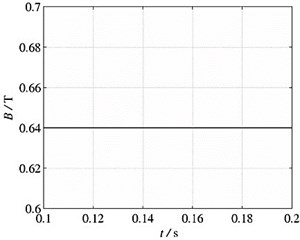a)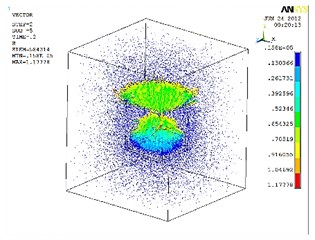b)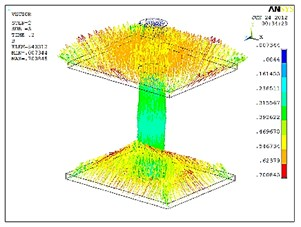c)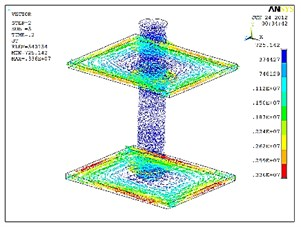d)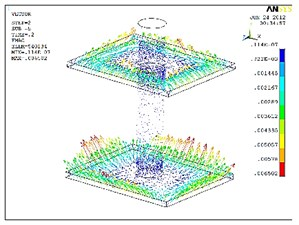e)

## 4. Optimization design of the ECD

The purpose of this section is to experimentally determine the influence of the geometry of the ECD’s main parts on the damping coefficient based on 3D transient analysis method. The magnetic force exerted on the magnet depends on the portion of the eddy current that is within the magnetic field created by the magnet.

### 4.1. Optimization of the magnet thickness

This section focuses on the influence of magnet thickness on the damping force, which can be targeted to select an optimal magnet size in thickness direction. It is very important for lightweight design of the damper. The damper is in the equilibrium position, and the speed is 0.1 m/s.

Calculation results were shown in Fig. 13 (specific data were listed in Table 6). It was observed that when the magnet thickness was smaller than a value, about 30 mm, the damping force increased with the magnet thickness. However, when the magnet thickness increased to about 30 mm, the increase of damping force was not very obvious, and it was larger than 34 mm, the damping force would decrease with the magnet thickness. In addition, compared the damping force of the upper plate with the down one, it found that the former was less than the latter in all conditions, which proving the importance of the down plate. When the magnet thickness increased, the damping force of the upper plate increased slowly, but the change of the down plate was obvious in the style of effect of magnet thickness on damping force (overall). Therefore, the damping force of the down plate was the most significant factor on the overall. According to the discussion above and the requirement of overall size, the magnet thickness was determined as 34 mm.

Fig. 13Effect of magnet thickness on damping force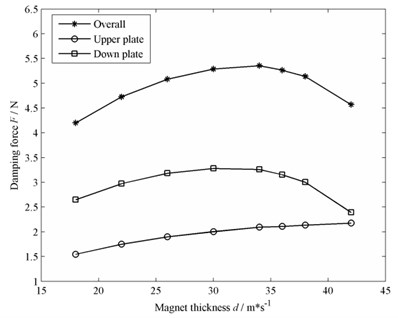Table 6The damping force (N) with the change of the magnet thickness (mm)

 Magnet thickness 42 38 36 34 30 26 22 18 Overall 4.5705 5.13941 5.26509 5.35679 5.28806 5.08339 4.72774 4.19977 Upper plate 2.17638 2.13507 2.11045 2.09482 2.0059 1.89975 1.75166 1.54842 Down plate 2.39412 3.00434 3.15464 3.26197 3.28216 3.18364 2.97607 2.65135

### 4.2. Optimization of the copper plate thickness

To determine the relationship between damping force and the copper plate thickness, several ECD models with different copper plate thickness were analyzed through 3D transient analysis. Fig. 14 showed the simulation results for the eddy current damping system. From the figure, it could be seen the change of damping force when the two copper plates thickness increased from 1 mm to 6 mm. Specific data were listed in Table 7. The copper plate thickness had a significant effect on the damping force, especially when the thickness was 5 mm, the damping force rapidly increased to 9.53841 N, which was larger than the result of 1 mm. However, if the plate thickness continued to increased, the damping force wouldn’t increase a lot. And when the plate became thicker, the overall weight of the ECD must be affected. Therefore, the copper plate was determined as 5 mm.

Fig. 14Effect of copper plate thickness on damping force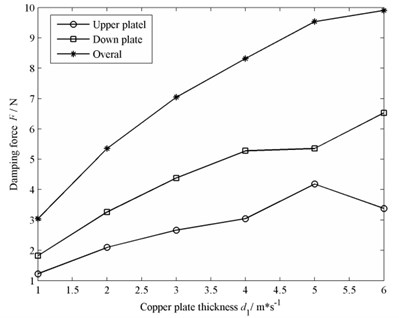Table 7The damping force (N) with the change of the copper plate thickness (mm)

 Copper plate thickness 1 2 3 4 5 6 Upper plate 1.22615 2.09482 2.66561 3.04244 4.18268 3.37742 Down plate 1.81718 3.26197 4.38039 5.27708 5.35573 6.52787 Overall 3.04333 5.35679 7.046 8.31952 9.53841 9.90529

Now that the improvements in the model had been confirmed, the performance of the enhanced ECD could be demonstrated.

Fig. 15 showed the results of optimized model test. The improved ECD model that utilizes optimum geometry sizes had a damping force as large as 12.4079 N.

Fig. 153D transient analysis results of the optimized model: a) magnetic field distribution of the two copper plates, b) eddy current distribution of the two copper plates, c) Lorentz force distribution of the two copper plates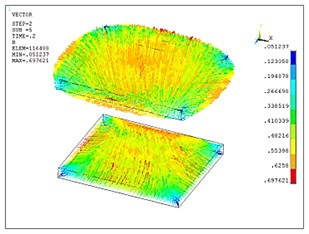a)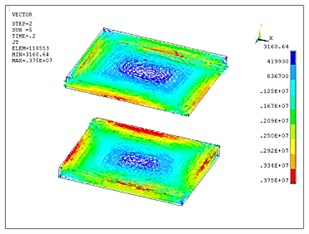b)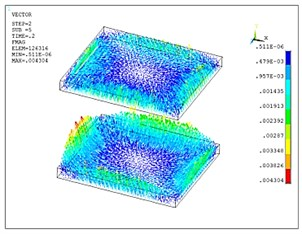c)

The damping coefficient for the whole ECD with four copper conductors can be obtained from the damping force:

15
$c=\frac{F}{v}.$

Therefore, the damping coefficient was calculated as 124.079 Ns/m. The results demonstrated that this novel ECD has high damping performance with simple structure. It was applicable in various vibration isolation systems such as spacecraft suspension systems and structure vibration isolation.

## 5. Conclusions

In this paper, a passive magnetic damper system was developed by using the eddy current damping effect. The proposed ECD utilized a stationary permanent magnet and two conductive copper plates. A theoretical model of the proposed system was constructed by using the motional eddy current estimation and the transformer eddy current contribution. The magnetic flux, and eddy current damping force were analytically calculated and validated by FE results. To optimize the design, simulations were conducted and the design parameters were evaluated. When the optimized ECD model was established, simulations were conducted to verify the accuracy of the theoretical model. The developed analytical model was used to design high-performance dampers for a variety of applications. The damping characteristic of the proposed system can be easily changed by either re-positioning the conductors or the permanent magnet, or choosing the appropriate conductor size and the air-gap distance between the magnets.

Because the damper is of passive nature, it is robust to parameter changes, requires no additional energy, and is easy to apply to the structure. Furthermore, the damper developed in this paper is a noncontact damper, thus allowing significant damping to be added while allowing the other properties and dynamics of the structure to be unaffected by its addition to the system. This point is of significant importance to the many structures that are designed with specific key parameters in place, through which the addition of a damping scheme such as constrained layer damping would change. The ECD developed in this paper utilized two copper plates and was shown to significantly outperform previously designed systems that utilize only one plate, and it is suitable for different vibrational structures for high accuracy and simple implementation. The proposed ECD can be modified in terms of size, material, and topological design for different applications. Future work might involve extending the magnetic damper design for precision optical instruments damper systems installed in space shuttle, since the damper is oil free, inexpensive, requires no external power, and is simple to manufacture. The manufacture of the prototype of ECD is ongoing, and refinement and testing work will be added.